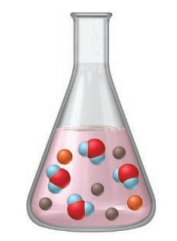# Problem: The flask represents the products of the titration of 25 mL of sulfuric acid with 25 mL of sodium hydroxide.What are the molarities of the acid and the base?

###### FREE Expert Solution
84% (284 ratings)
###### Problem Details

The flask represents the products of the titration of 25 mL of sulfuric acid with 25 mL of sodium hydroxide.What are the molarities of the acid and the base?

Frequently Asked Questions

What scientific concept do you need to know in order to solve this problem?

Our tutors have indicated that to solve this problem you will need to apply the Solution Stoichiometry concept. You can view video lessons to learn Solution Stoichiometry. Or if you need more Solution Stoichiometry practice, you can also practice Solution Stoichiometry practice problems.

What textbook is this problem found in?

Our data indicates that this problem or a close variation was asked in Chemistry: The Molecular Nature of Matter and Change - Silberberg 8th Edition. You can also practice Chemistry: The Molecular Nature of Matter and Change - Silberberg 8th Edition practice problems.